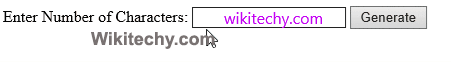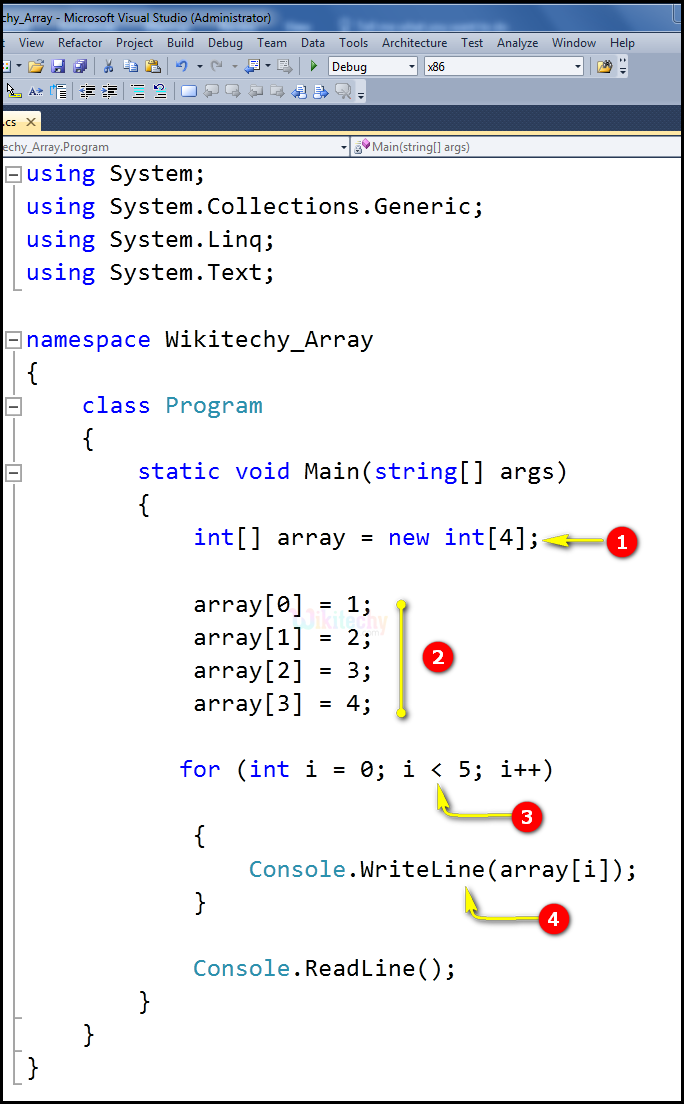# C# Random - c# - c# tutorial - c# net

## What is Random Number in C# ?

• In C#, the Random class defined in the .NET Framework class library provides functionality to generate random numbers.
• This method is used to generate random string.• The Random class constructors have two overloaded forms. It takes either no value or it takes a seed value. The Random class has three public methods
• Next,
• NextBytes, and
• NextDouble.• ## Next:

The Next method is used to returns a random number.
• ## NextBytes:

NextBytes method returns an array of bytes filled with random numbers.
• ## NextDouble:

The next double method returns a random number between 0.0 and 1.0.

• ## datatype:

Datatype is used to specify the type of elements in the array. And [ ] specifies the rank of the array. The rank specifies the size of the array.
• ## arrayName:

ArrayName specifies the name of the array.

## Code Explanation:• int[] array = new int; We declare and initialize a numerical array. And we declare an array which contains 4 elements. All elements are integers.
• We initialize the array with some data. This is assignment initialization. The indexes are in the square brackets. Array  is going to be the first element of the array. Array  is going to be the second element of the array, Array  is going to be the third element of the array, Array  is going to be the fourth element of the array.
• Here for (int i = 0; i < 5; i++) specifies integer i = 0, which means the expression, (I < 5), is true. Therefore, the statement is executed, and i gets incremented by 1 and becomes 4.
• Here we can declare and initialize an array in one statement array[i].

## Sample C# examples - Output :• Here in this output the 1,2,3,4 will be printed until it reaches the less than "5" array elements.